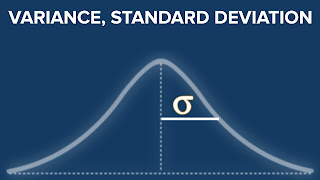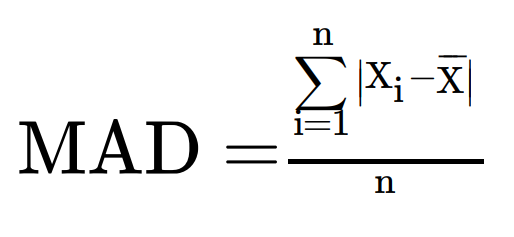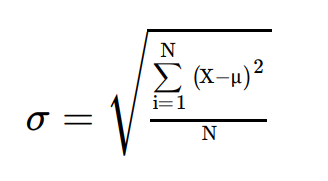As usual, My colleague "Jose" surprised "Austin Lasseter" (our Instructor) with a good question, and make the class stop for a while.

This time Jose made a shock for all of us when he notes that the calculation of the "mean absolute error" is so close to the calculation of the "standard deviation". then he asks "why not using standard deviation?" he means Why we do not use standard divination instead to mean absolute error in Regression Models?

Actually, when I focused more, I found something really fantastic, the "mean absolute error" which we study in Data science to make a Regression is the same the "Average Deviation"  or we commonly called it "The mean absolute deviation (MAD)" which we use it to measure the volatility of returns of the portfolio (in investment).And in the investment, we prefer to use the standard deviation rather than "The mean absolute deviation (MAD)", as both measures the volatility of returns from investment funds or strategies because it can help measure volatility. Higher volatility is generally associated with a higher risk of losses, so investors want to see higher returns from funds that generate higher volatility.But why we prefer to use the standard deviation rather than "The mean absolute deviation (MAD)" in investment and finance life? the answer is the same answer for Jose's question!!

Generally, according to mathematicians, when a data set is of normal distribution — that is, there aren't many outliers — the standard deviation is the preferable gauge of variability. But when there are large outliers, the standard deviation will register higher levels of dispersion, or deviation from the center, than the mean absolute deviation or mean absolute error.

So in Investment when we try to measure the risk we assume that the data is normally distributed because the volatility of data is repeated so we do not have many outliers (this is the neutral of this data in general).

But in Regression Models, you do not know the neutral of the data you work with, if you would like to use the standard deviation, go-ahead, but make sure first that the data is normally distributed.

1.Nice Article, Keep it up…
Ventura County Real Estate Investors Association is very famous Investors Clubs in Ventura County and everyone who is planning their in future in real estate must visit them and take mentoring programs from them which will definitely guide them at each end every step.

It is important for me to know your opinion in this contact.